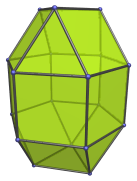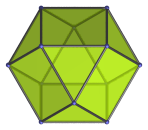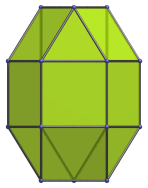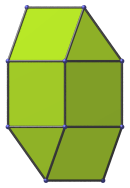# The Elongated Triangular Gyrobicupola

The elongated triangular gyrobicupola is the 36th Johnson solid (J36). It has 18 vertices, 36 edges, and 20 faces (8 equilateral triangles and 12 squares).The elongated triangular gyrobicupola can be constructed by attaching two triangular cupolae to a hexagonal prism, or equivalently, by inserting a hexagonal prism between the two halves of a cuboctahedron (as a triangular gyrobicupola). The gyro- in the name refers to how the orientation of the top and bottom triangles are rotated with respect to each other. If the top and bottom triangles are aligned with respect to each other, the elongated triangular orthobicupola (J35) is produced instead.

## Projections

Here are some views of the elongated triangular gyrobicupola from various angles:

Projection DescriptionTop view.Front view.Side view.

## Coordinates

The Cartesian coordinates of the elongated triangular gyrobicupola with edge length 2 are:

• (±1, −1/√3, 1+√(8/3))
• (0, 2/√3, 1+√(8/3))
• (±1, ±√3, ±1)
• (±2, 0, ±1)
• (±1, 1/√3, −(1+√(8/3)))
• (0, −2/√3, −(1+√(8/3)))

Last updated 16 May 2018.